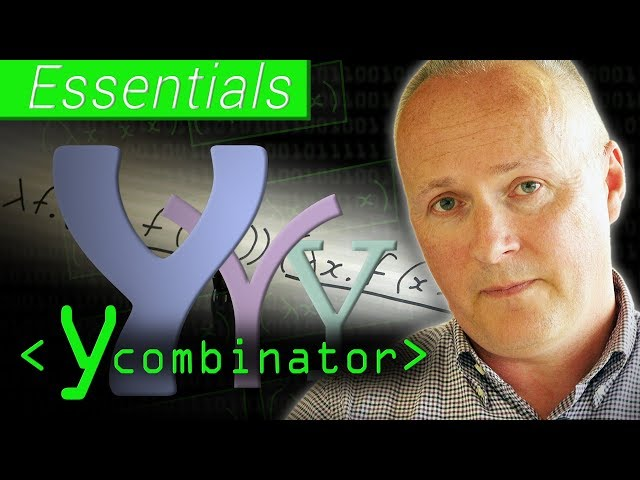# Functional Programming's Y Combinator - ComputerphileTLDR The Y combinator is a powerful concept that enables recursion in programming languages without built-in recursion, and it serves as a metaphor for a startup incubator's mission of supporting companies by running other programs.

## Key insights

• 🔄
The Y combinator allows for recursion in a language that doesn't have any recursion or looping mechanism at all, providing a clever solution to this limitation.
• 🔄
Functional programming allows for elegant and concise solutions, such as the factorial function, by breaking down complex problems into smaller, self-referential components.
• 🤔
The encoding of True and False as functions in functional programming allows for the definition of any other logical function, highlighting the flexibility and power of this approach.
• 🔄
The concept of self application in the Lambda Calculus allows for looping or recursion in a language that doesn't have built-in support for it.
• 🔄
The Y Combinator function demonstrates the concept of self-application, where the input is substituted for the function itself, resulting in a loop that never progresses.
• 🧩
The recursive function "rec" can be used to encode any other recursive function, making it a powerful tool in functional programming.
• 🧠
If we can encode general recursion in the Lambda Calculus, we can do everything.
• 🌐
Alonzo Church, the mathematician who invented the Lambda Calculus, was interested in understanding the notion of a function.

## Q&A

• What is the Y combinator?

The Y combinator is a concept that enables recursion in programming languages without built-in recursion or looping mechanisms.

• How does the factorial function work?

The factorial function takes a number and recursively multiplies it by the factorial of the number minus 1 until it reaches 1.

• What is the Lambda Calculus?

The Lambda Calculus is a minimal language for defining functions using variables, lambda notation, and function application.

• How is recursion encoded in the Lambda Calculus?

Recursion is encoded in the Lambda Calculus through self application, where a function is applied to itself, allowing for repetition of a behavior.

• What is the Y combinator's significance in Computer Science?

The Y combinator is a fundamental idea in Computer Science that allows for recursion in a language that lacks built-in recursion, and it serves as a metaphor for a startup incubator's mission of supporting companies by running other programs.

## Timestamped Summary

• 📺
00:00
The Y combinator is one of the most interesting and weirdest ideas in Computer Science, and the speaker will discuss it in this video.
• 📝
00:33
The Y combinator enables recursion in languages without recursion or looping mechanisms, allowing for the calculation of factorial by multiplying a number with the factorial of its predecessor until reaching the base case.
• 📝
03:09
The Lambda Calculus is a minimal language for defining functions using variables, lambda notation, and function application, with logical values True and False encoded as functions that choose between two different things.
• 📚
04:39
Recursion in Lambda Calculus is achieved through self application, enabling the repetition of behavior in a language that lacks native support for looping.
• 🔄
06:54
A function that takes an input and produces two copies of it side by side can create a loop where the output continuously returns to the same point.
• 📝
08:01
The function "rec" in functional programming applies a given function infinitely, making it the most general pattern of recursion that can be used to encode any other recursive function.
• 📝
09:09
Define loop as a recursive function to define the factorial function, and with the ability to encode general recursion in the Lambda Calculus, we can accomplish anything.
• 💡
10:31
The Y combinator is a powerful concept that enables recursion in programming languages without built-in recursion, and it serves as a metaphor for a startup incubator's mission of supporting companies by running other programs.Report the articleThank you for the feedback